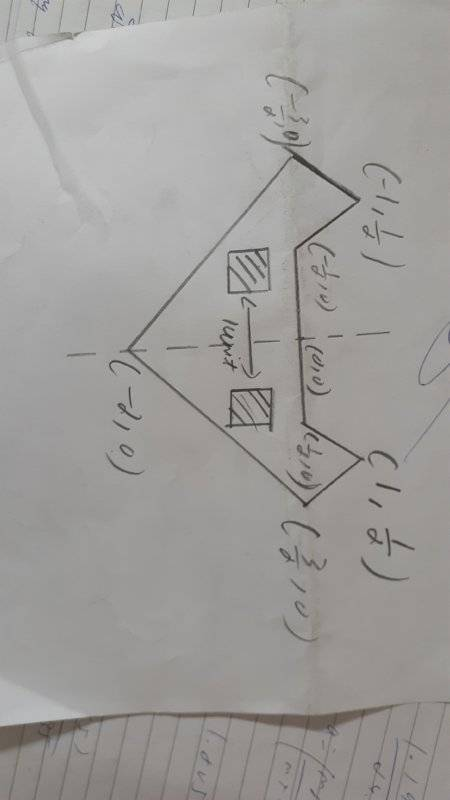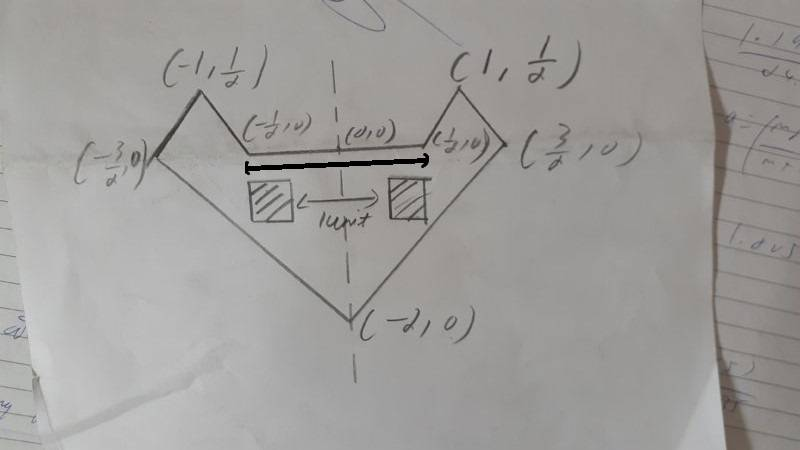# Surface integrals to calculate the area of this figure

• VVS2000

#### VVS2000

Homework Statement
My homework is to find the area of the following figure(leaving those two squares)
Relevant Equations
The distance between those two squares is one unit. Please do tell if the figure is not descriptive enoughI can find the area of the triangles but can't solve the squares for some reason

Last edited by a moderator:
Sry for not including the equations section, I don't know how to type integrals here

Sry for not including the equations section, I don't know how to type integrals here
Are you sure you need integration for this?

Well the assignmet was clear of me using integration

And the squares are of 1/2 unit length

Well the assignmet was clear of me using integration
You can, course, use integration to calculate the area of a square or a triangle - just for practice.See the LaTeX tutorial at the top of the page under INFO, Help.